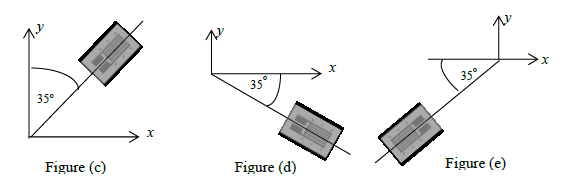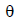# 9.2 Strain Gages

1. In Figure (a) strain gages 1 and 2 recorded strains of 700 and -800 micro, respectively. The normal strains are
2. In Figure (b) strain gages 1 and 2 recorded strains of 700 and -800 micro, respectively. The normal strains are
3.The anglethat can be substituted in the strain transformation Equation 9.5  relating the strain gage reading to strains in Cartesian coordinates for Figure (c) is
4. The anglethat can be substituted in the strain transformation Equation 9.5  relating the strain gage reading to strains in Cartesian coordinates for Figure (d) is
5. The anglethat can be substituted in the strain transformation Equation 9.5  relating the strain gage reading to strains in Cartesian coordinates for Figure (e) is
6.A point in plane stress has the Mohr circle for strain shown in Figure (f). The modulus of elasticity of the material is E = 10,000 ksi and Poisson’s ratio is 0.25. The maximum shear strain is
7. A point in plane stress has the Mohr circle for strain shown in Figure (g). The modulus of elasticity of the material is E = 10,000 ksi and Poisson’s ratio is 0.25. The maximum shear strain is
8. A point in plane stress has the Mohr circle for strain shown in Figure (h). The modulus of elasticity of the material is E = 10,000 ksi and Poisson’s ratio is 0.25. The maximum shear strain is
9. In plane strain there are two principal strains, but in plane stress there are three principal strains.
10. The principal coordinate axis for stresses and strains is always the same, irrespective of the stress–strain relationship.

Do you have any comments or suggestions? Please send them to author@madhuvable.org